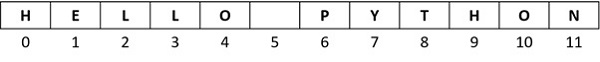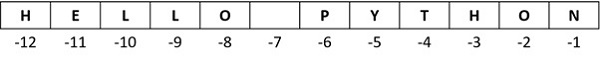# Python Slicing Strings

In Python, a string is an ordered sequence of Unicode characters. Each character in the string has a unique index in the sequence. The index starts with 0. First character in the string has its positional index 0. The index keeps incrementing towards the end of string.

If a string variable is declared as var="HELLO PYTHON", index of each character in the string is as follows −Python allows you to access any individual character from the string by its index. In this case, 0 is the lower bound and 11 is the upper bound of the string. So, var returns H, var returns P. If the index in square brackets exceeds the upper bound, Python raises IndexError.

```>>> var="HELLO PYTHON"
>>> var
'H'
>>> var
'Y'
>>> var
'N'
>>> var
Traceback (most recent call last):
File "<stdin>", line 1, in <module>
IndexError: string index out of range
```

One of the unique features of Python sequence types (and therefore a string object) it has a negative indexing scheme also. In the example above, a positive indexing scheme is used where the index increments from left to right. In case of negative indexing, the character at the end has -1 index and the index decrements from right to left, as a result the first character H has -12 index.Let us use negative indexing to fetch N, Y, and H characters.

```>>> var[-1]
'N'
>>> var[-5]
'Y'
>>> var[-12]
'H'
>>> var[-13]
Traceback (most recent call last):
File "<stdin>", line 1, in <module>
IndexError: string index out of range
```

Once again, if the index goes beyond the range, IndexError is encountered.

We can therefore use positive or negative index to retrieve a character from the string.

```>>> var, var[-12]
('H', 'H')
>>> var, var[-5]
('Y', 'Y')
>>> var, var[-1]
('N', 'N')
```

In Python, string is an immutable object. The object is immutable if it cannot be modified in-place, once stored in a certain memory location. You can retrieve any character from the string with the help of its index, but you cannot replace it with another character. In our example, character Y is at index 7 in HELLO PYTHON. Try to replace Y with y and see what happens.

```var="HELLO PYTHON"
var="y"
print (var)
```

It will produce the following output

```Traceback (most recent call last):
File "C:\Users\users\example.py", line 2, in <module>
var="y"
~~~^^^
TypeError: 'str' object does not support item assignment
```

The TypeError is because the string is immutable.

Python defines ":" as string slicing operator. It returns a substring from the original string. Its general usage is −

```substr=var[x:y]
```

The ":" operator needs two integer operands (both of which may be omitted, as we shall see in subsequent examples). The first operand x is the index of the first character of the desired slice. The second operand y is the index of the character next to the last in the desired string. So var(x:y] separates characters from xth position to (y-1)th position from the original string.

```var="HELLO PYTHON"

print ("var:",var)
print ("var[3:8]:", var[3:8])
```

It will produce the following output

```var: HELLO PYTHON
var[3:8]: LO PY
```

Negative indexes can also be used for slicing.

```var="HELLO PYTHON"
print ("var:",var)
print ("var[3:8]:", var[3:8])
print ("var[-9:-4]:", var[-9:-4])
```

It will produce the following output

```var: HELLO PYTHON
var[3:8]: LO PY
var[-9:-4]: LO PY
```

Both the operands for Python's Slice operator are optional. The first operand defaults to zero, which means if we do not give the first operand, the slice starts of character at 0th index, i.e. the first character. It slices the leftmost substring up to "y-1" characters.

```var="HELLO PYTHON"
print ("var:",var)
print ("var[0:5]:", var[0:5])
print ("var[:5]:", var[:5])
```

It will produce the following output

```var: HELLO PYTHON
var[0:5]: HELLO
var[:5]: HELLO
```

Similarly, y operand is also optional. By default, it is "-1", which means the string will be sliced from the xth position up to the end of string.

```var="HELLO PYTHON"
print ("var:",var)
print ("var[6:12]:", var[6:12])
print ("var[6:]:", var[6:])
```

It will produce the following output −

```var: HELLO PYTHON
var[6:12]: PYTHON
var[6:]: PYTHON
```

Naturally, if both the operands are not used, the slice will be equal to the original string. That's because "x" is 0, and "y" is the last index+1 (or -1) by default.

```var="HELLO PYTHON"
print ("var:",var)
print ("var[0:12]:", var[0:12])
print ("var[:]:", var[:])
```

It will produce the following output

```var: HELLO PYTHON
var[0:12]: HELLO PYTHON
var[:]: HELLO PYTHON
```

The left operand must be smaller than the operand on right, for getting a substring of the original string. Python doesn't raise any error, if the left operand is greater, bu returns a null string.

```var="HELLO PYTHON"
print ("var:",var)
print ("var[-1:7]:", var[-1:7])
print ("var[7:0]:", var[7:0])
```

It will produce the following output

```var: HELLO PYTHON
var[-1:7]:
var[7:0]:
```

Slicing returns a new string. You can very well perform string operations like concatenation, or slicing on the sliced string.

```var="HELLO PYTHON"

print ("var:",var)
print ("var[:6][:2]:", var[:6][:2])

var1=var[:6]
print ("slice:", var1)
print ("var1[:2]:", var1[:2])
```

It will produce the following output

```var: HELLO PYTHON
var[:6][:2]: HE
slice: HELLO
var1[:2]: HE
```